# Custom Error Messages in Data Validation in Microsoft Excel 2010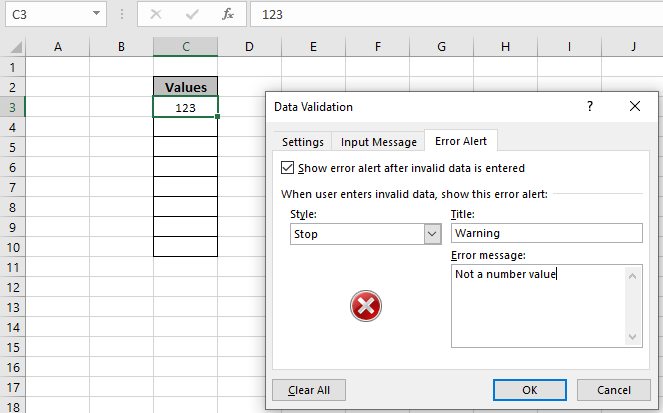In this article, we will learn How to give the error messages in Data Validation.

Scenario:

Data validation tool restricts the user to input data to ensure its accuracy and consistency in Excel. It guides the user to input the type of option which is necessary for your worksheet.

Here the problem is, we need to check if the cell has a number or not i.e. Excel cells only accept number values. If you want to learn more about Data validation in Excel, follow the link. Here we will just consider the problem, allow values only with no number.

How to solve the problem?

Here we have a task that allows entries with data type number only. The use of functions and a tool will help us get through the problem.

The formula be used in Data Validation tool. The formula checks the condition and returns True or False. Here the True means that the punctuation doesn't exist, so the tool accepts the value.

Generic formula:

 =ISNUMBER(cell_ref)

Explanation:

ISNUMBER function checks the value argument and returns True, if number or False.

Example :

All of these might be confusing to understand. So, let's test this formula via running it on the example shown below. Here we will apply a data validation on the cell to allow the user to input any value which is a number. Below is the formula to apply.

Use the formula:

 =ISNUMBER(C3)=0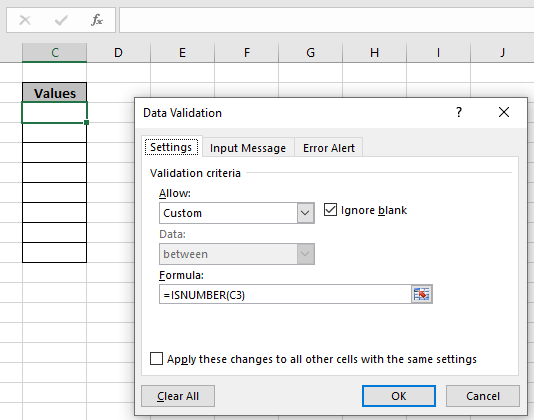Here you noticed, Select Custom from Allow method list which allows you to use the formula in cell. Click Ok to apply.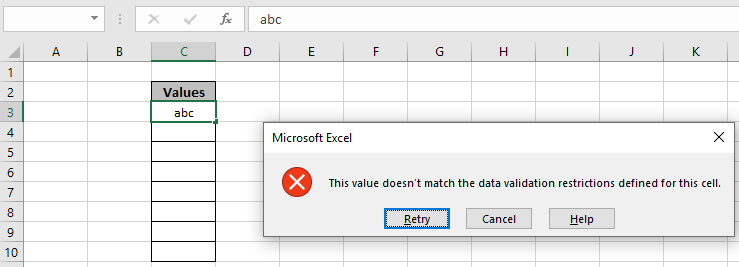We tried typing the text value as we enter a text value, it throws an error "This value doesn't match the data validation restrictions defined for this cell".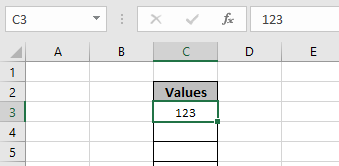Now, we tried the number value in the cell and the cell accepts the number value as shown in the above image. You can also customize the error message and alert message to let users know what values are prohibited. Edit alert and message as shown below.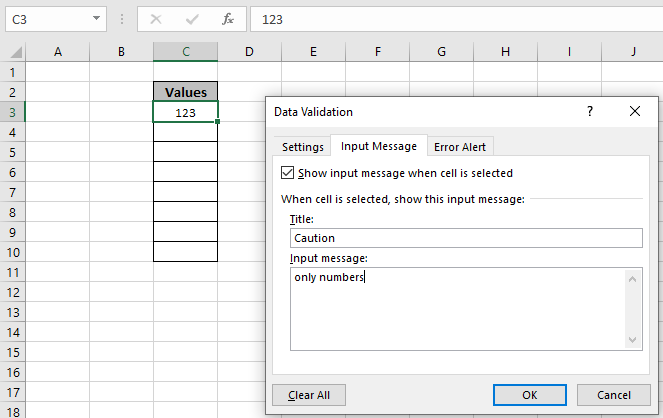Input Alert message be typed in Input Message tab as shown in above image.An Error Alert message be used in the Error Alert tab. Now click OK to get these changes.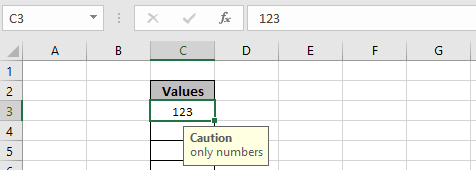As you can see the Caution alert in the yellow box hanging below C3 cell. Now you can copy the Data validation format to the rest of the required cell using Ctrl + D.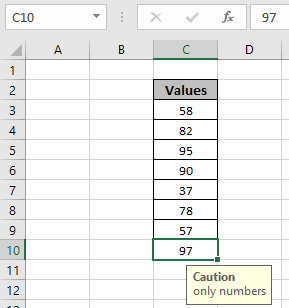As you can see, now these cells can be used to allow numbers only. You can use a formula to allow text value only i.e. =ISTEXT(cel_ref). You can use any formula that returns True or False in the formula bar of the data validation tool.

Another Example

Here we have some formula in data validation tool and we need to custom or change error message whenever cell reject any value in Excel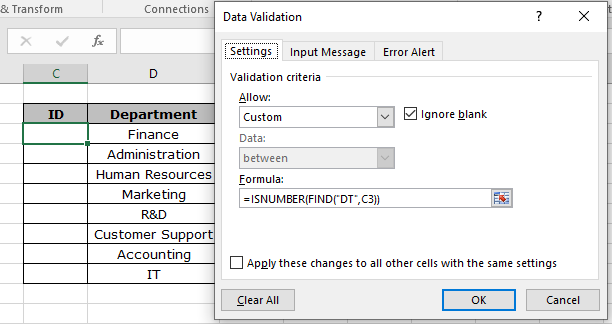Now when I enter the value as shown below. A default error generates.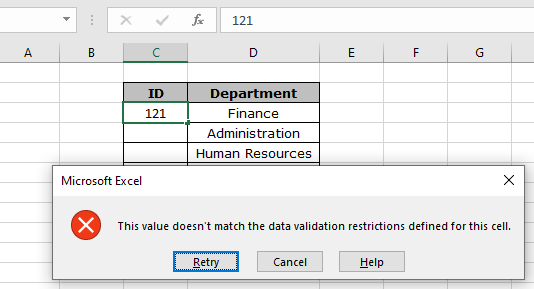As you can see an error is generated "This value doesn't match the data validation restrictions defined for this cell".

But now the problem is how the user know what restrictions are there and what type of value can the cell accept.

So for this, we will customize some changes as shown below.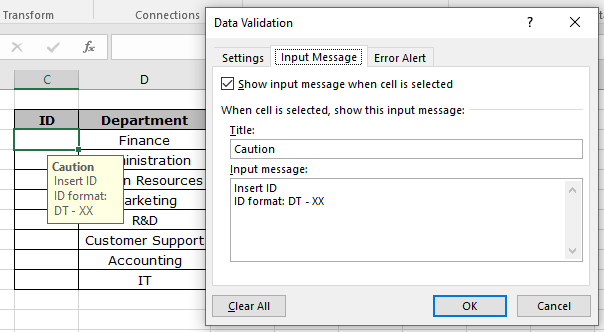In Input Message option, type the details under Title & Input Message box as shown above.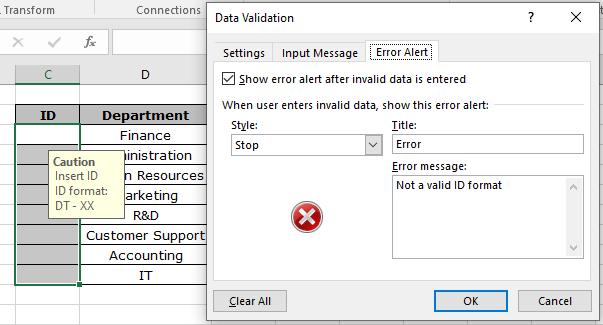In Error alert option, type the details under Title & Error Message box as shown above.

Click Ok.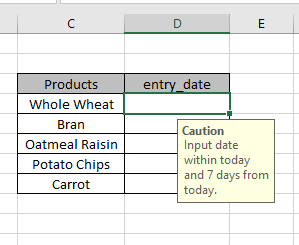A message is displayed when the cell is selected And an error message is displayed as shown below.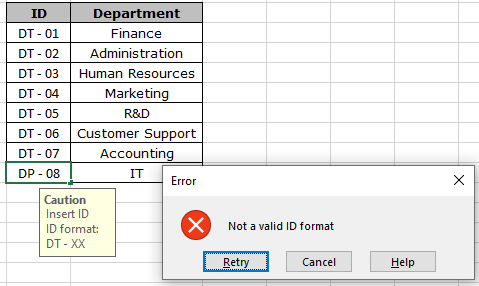Now fill the right answers, to complete the table.

To copy the data validation rule to the required cells use the drag down or drag right option in excel.As you can see, our table is complete. Now its user friendly and informative as well.

Here are all the observational notes regarding using the formula.

Notes:

1. Apply the data validation tool to restrict the user to input invalid value, which doesn't satisfy the condition.
2. Data validation tool can work with numbers and text both.

Hope this article about How to validate only numbers in cells in Excel is explanatory. Find more articles on data validation tools with formulas here. If you liked our blogs, share it with your friends on Facebook. And also you can follow us on Twitter and Facebook. We would love to hear from you, do let us know how we can improve, complement or innovate our work and make it better for you.

Related Articles:

Data Validation in Excel : Data Validation is a tool used to restrict users to input value manually in cell or worksheet in Excel. It has a list of options to choose from.

Way to use Vlookup function in Data Validation : Restrict users to allow values from the lookup table using Data validation formula box in Excel. Formula box in data validation allows you to choose the type of restriction required.

Restrict Dates using Data Validation : Restrict user to allow dates from a given range in cell which lays within Excel date format in Excel.

How to give the error messages in Data Validation : Restrict users to customize input information in the worksheet and guide the input information through error messages under data validation in Excel.

Create Drop Down Lists in Excel using Data Validation : Restrict users to allow values from the drop down list using Data validation List option in Excel. List box in data validation allows you to choose the type of restriction required.

Popular Articles :

How to use the IF Function in Excel : The IF statement in Excel checks the condition and returns a specific value if the condition is TRUE or returns another specific value if FALSE.

How to use the VLOOKUP Function in Excel : This is one of the most used and popular functions of excel that is used to lookup value from different ranges and sheets.

How to Use SUMIF Function in Excel : This is another dashboard essential function. This helps you sum up values on specific conditions.

How to use the COUNTIF Function in Excel : Count values with conditions using this amazing function. You don't need to filter your data to count specific values. Countif function is essential to prepare your dashboard.

Terms and Conditions of use

The applications/code on this site are distributed as is and without warranties or liability. In no event shall the owner of the copyrights, or the authors of the applications/code be liable for any loss of profit, any problems or any damage resulting from the use or evaluation of the applications/code.